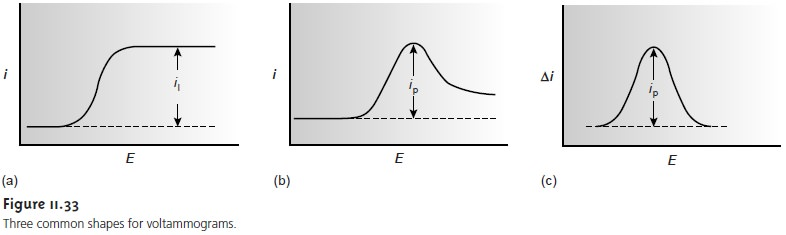Home | | Modern Analytical Chemistry | Shape of Voltammograms

# Shape of Voltammograms

The shape of a voltammogram is determined by several experimental factors, the most important of which are how the current is measured and whether convection is included as a means of mass transport.

Shape of Voltammograms

The shape of a voltammogram is determined by several experimental factors, the most important of which are how the current is measured and whether convection is included as a means of mass transport. Despite an abundance of different voltam- metric techniques, only three shapes are common for voltammograms (Figure 11.33).The voltammogram in Figure 11.33a is characterized by a current that increases from the background residual current to a limiting current at potentials at which the analyte is oxidized or reduced. Since the magnitude of a faradaic current is in- versely proportional to δ  (equation 11.33), a limiting current implies that the thick- ness of the diffusion layer remains constant. The simplest method for obtaining a limiting current is to stir the solution (Figure 11.32), which can be accomplished with a magnetic stir bar, or by rotating the electrode. Voltammetric techniques that include convection by stirring are called hydrodynamic voltammetry. When con- vection is absent, the thickness of the diffusion layer increases with time (Figure 11.31), resulting in a peak current in place of a limiting current (Figure 11.33b).

In the voltammograms in Figures 11.33a and 11.33b, the current is monitored as a function of the applied potential. Alternatively, the change in current following a change in potential may be measured. The resulting voltammogram, which is shown in Figure 11.33c, also is characterized by a peak current.

Study Material, Lecturing Notes, Assignment, Reference, Wiki description explanation, brief detail
Modern Analytical Chemistry: Electrochemical Methods of Analysis : Shape of Voltammograms |